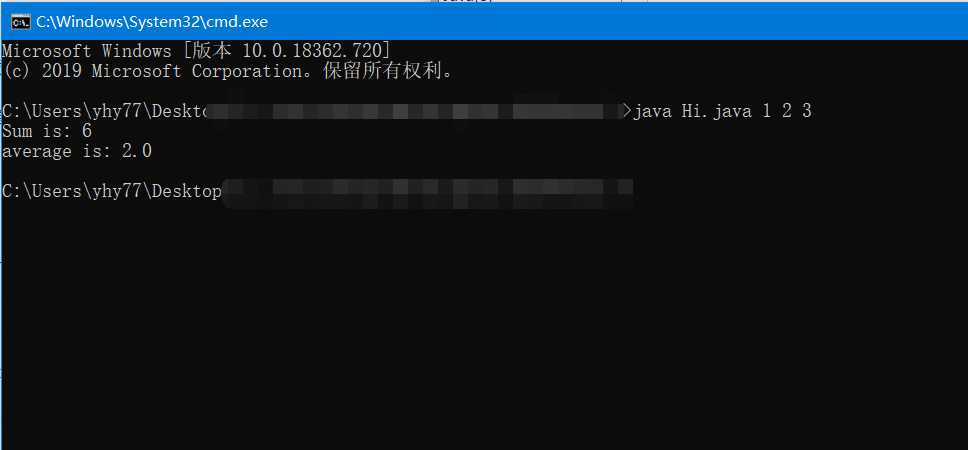# Java中的（String args[]）

1. DOS下运行

```class Hi {
public static void main (String a[]) {
int sum = 0;

for (int i = 0; i < a.length; i++) {
sum += Integer.parseInt(a[i]);
}

System.out.println("Sum is: "+sum);
System.out.println("average is: "+(float)sum / (float)a.length);}
}```2. 可视化编辑器下运行（以IDEA为例）

Java中的（String args[]）

(0)
(0)